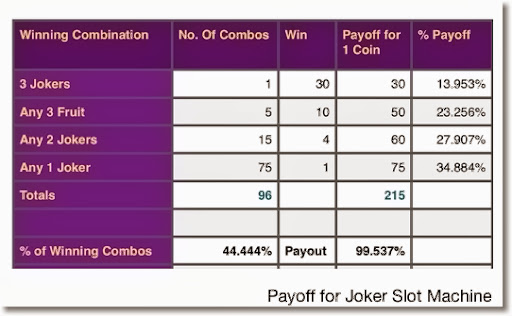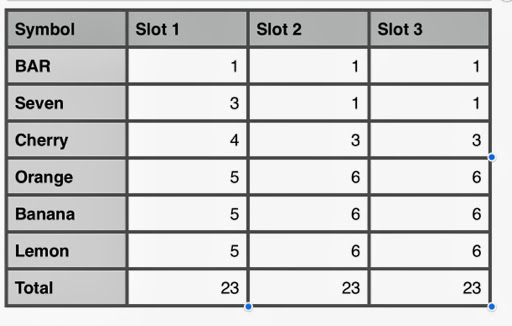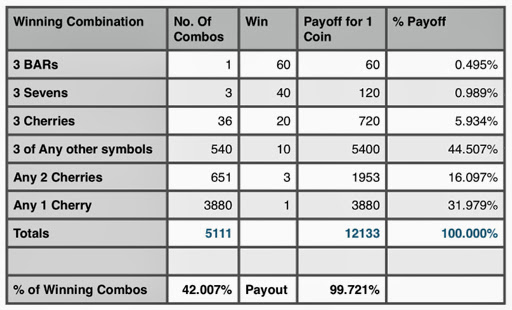## Tuesday, January 7, 2014

### Probability: Odds of Winning at Slot Machines

The Odds of Hitting it Big

The number of possible combinations is fairly easy to calculate. You multiply the number symbols each slot has together. For example for a three slot machine with six symbols a piece, the number possible combinations is 6 × 6 × 6 = 216. Although I have never played on one (knowingly), you could have a slot machine were each slot itself has a different amount of symbols.

Calculating the probability of winning on a slot machine is fairly simple. The odds of getting a certain combination is:

(number of winning combinations)/(number of possible combinations)

Different winning combinations offer different playoffs. The harder the combination, the bigger the reward. In gambling, the term payout percent is used when describing how "lucrative" the slot machine is. In general it is calculated like this:

Σ(winning combination_k * payoff_k)/(number of possible combinations)

Note that is different from the total of number of winning combinations over the number of possible combinations ratio. The payoff acts as a weight for each winning combination. (It is like weighted average, but not quite.)

Let us look at analyzing a pair of slot machines. For this blog entry, we will assume that the slot machine has one line and takes only one coin per roll.

Analyzing a Simple Slot Machine

The first machine has three slots. On each slot there is an apple, orange, lemon, banana, melon, and a Joker. The payouts are as follows:

(I) Three Jokers (Joker | Joker | Joker) pays 30 coins
(II) Any Three of the same fruit (example: Apple | Apple | Apple) pays 10 coins
(III) Any Two Jokers pays 4 coins
(IV) Any One Joker pays 1 coin (break even)

Number of Possible Combinations: 6 × 6 × 6 = 216

Number of Winning Combinations (see above):

(I): There is only one configuration for this: three Jokers.

(II): Three of a kind that isn't a Joker. One the first slot, you can get any of the five fruit (apple, orange, lemon, banana, melon). Once that fruit is selected, that fruit has to match on the next two slots. So, there are 5 such combinations: three apples, three oranges, three lemons, three bananas, and three lemons.

(III): Jokers can appear any two slots: slots 1 and 2, slots 1 and 3, and slots 2 and 3. The other slot must contain fruit. Hence the total number of winning combinations possible is 1 × 1 × 5 + 1 × 5 × 1 + 5 × 1 × 1 = 15.

(IV): Jokers can appear in any slot, the other two slots must have fruit. In this instance, it does not matter if the other two slots match. The number of combinations is 1 × 5 × 5 + 5 × 1 × 5 + 5 × 5 × 1 = 75.

There are a total of 1 + 5 + 15 + 75 = 96 winning combinations. The table below shows the calculation of slot's payout.Calculating the payoff percentage:

(1 × 30 + 5 × 50 + 15 × 4 + 75 × 1)/(6 × 6 × 6)
= 215/216
≈ 0.99537

The payoff percentage is 99.537%. That is pretty good in terms of slot machines. It could be a little misleading given that the harder combinations are given more weight.

Had everything paid equally, only 96/216, or about 44.444% of the combinations would win. I doubt no one would play a slot machine with less than a 50% chance of spinning a winning if there was no added incentive, such as a richer payoff.

A More Complex Slot Machine

We are still at one line, three slots. Here is the slot distribution:There are 23 symbols per slot. So the number of possible combinations is 23 × 23 × 23 = 12,167.

Also note that the non-equal distribution of symbols on each slot. With the lack of BARs, cherries, and Sevens (7), it is obvious that getting winning combinations involving these three symbols offer higher playoffs.

The winning combinations, with payoffs are: (See the table above for the distribution of symbols)

(I) 3 BARs. Wins 60 coins. Number of winning combinations: 1

(II) 3 Sevens. Wins 40 coins. Number of winning combinations: 3 × 1 × 1 = 3

(III) 3 Cherries. Wins 20 coins.
Number of winning combinations: 4 × 3 × 3 = 36

(IV) 3 of any Fruit (3 Oranges, 3 Bananas, 3 Lemons)
Wins 10 coins.
Number of winning combinations:
For Oranges: 5 × 6 × 6 = 180
For Bananas: 5 × 6 × 6 = 180
For Lemons: 5 × 6 × 6 = 180
Total: 540

(V) Cherries in Any Two Slots:
Wins 4 coins.
Number of winning combinations:
Cherry, Cherry, Other: 4 × 3 × (23-3) = 240
Cherry, Other, Cherry: 4 × (23-3) × 3 = 240
Other, Cherry, Cherry: (23-4) × 3 × 3 = 171
Total: 651

(VI) Cherry in any one slot:
Wins 1 coin.
First Slot: 4 × 20 × 20 = 1600
Second Slot: 19 × 3 × 20 = 1120
Third Slot: 19 × 20 × 3 = 1120
Total: 3880

The following table calculates the payoff percentage for this machine:This second table has a payoff percentage of 99.721%. If everything paid 1 coin equally, it would drop to just over 42%.

For more complex slot machines (more slots and multiple lines), the use of a spreadsheet or other computer program will come to the aid of calculating payoff since the number of calculations grow with complexity.

Gamble responsibly!

Eddie

Source:
Slot Machine Math - Gambler's Bookcase
http://www.gamblersbookcase.com/Slots-Math.htm

This blog is property of Edward Shore. 2014